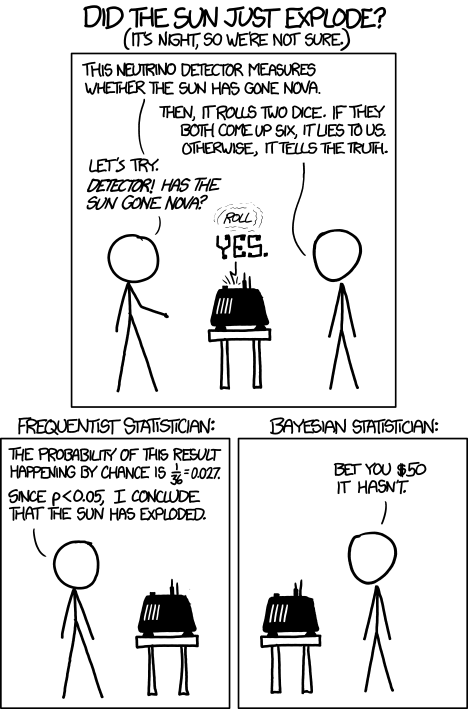## XKCD: Frequentists vs Bayesians

November 15, 2012

XKCD (an excellent web comic about computing and science) recently wrote a strip about the never-ending battle between Frequentists and Bayesians:First, I'd like to discuss what the comic means, or even what the heck a frequentist or a bayesian is anyway. But then, I'd like to respond to the central point of the comic from a frequentists point of view.

There are two common ways of interpreting probability. A frequentist defines probability as a percentage of times one obtains a result when repeating an experiment many times. Flipping a coin and getting heads has a 50% probability because if we flip a coin many times, we expect half of them to be heads. A bayesian sees probability as a degree of certainty. He would say that for any one flip, based on his knowledge of coins, he has a 50% certainty that the result will be heads.

The difference between the two seems subtle, but it means that the two camps have to make different types of statements about probability and data. Frequentists are limited to making statements about the probability of some result based on a model, where as Bayesians are able to make statements about the probability of models or parameters of models based on data.

So, what does all this mean in terms of the comic? On the left, the frequentist make a seemingly odd statement to determine if the sun has exploded or not. He points out that the probability of the machine saying "the sun has exploded" under the assumption that the sun has NOT really exploded is 0.027 (this is known as a p-value). Since the p-value is smaller than some threshold he set (5%), he concludes that the sun has exploded. p-value tests like this one essentially quantify the rate of an accidental discovery, and these are precisely the kinds of tests that experimental particle physicists used to claim discovery of the Higgs boson.

The bayesian on the other hand is able to take into account the probability that the sun has actually exploded. He knows a lot about the sun and its lifetime, and has used that knowledge to build up a prior probability that the sun has actually exploded. This prior is most likely extremely strong (hes VERY sure that the sun hasnt really gone nova yet), and so the result of the machine is not going to have much influence on his opinion as to whether the sun has exploded or not. The effect of the machine is to slightly change this opinion, but not by much.

So, at first glance, the frequentists interpretation seems wrong and his analysis seems nonsensical. Why would somebody take such a camp seriously when their methodology seems to fall apart under a simple thought experiment?

The resolution of this concern is the fact that the bayesian and the frequentists are really on unequal footing. The bayesians analysis is using the entire wealth of human knowledge about the Sun in the construction of his prior. But, contrary to popular belief, a frequentist too is able to take into account this accumulated information. He simply must do so in a way that is consistent with his interpretation of probability. He simply must do so in the context of data, as opposed to belief.

The real question is Why does the frequentists result seem so strange, and if its because we know the sun hasnt really exploded yet, then HOW do we know this? We have a certain amount of certainty that the sun hasnt exploded because we've made measurements, observations, and simulations that can constrain when the sun will explode. So, our full knowledge should take those measurements and data points into account.

In a Bayesian analysis, this is done by using those measurements to construct a prior (although, the procedure to turn measurements into a prior isn't well-defined: at some point there must be an initial prior, or else it's "turtles all the way down"). So, when the Bayesian uses his prior, he's really taking into account a lot of additional information that the frequentist's p-value analysis isn't privy to.

So, to remain on equal footing, a full frequentist analysis of the problem should include the same additional data about the sun exploding that is used to construct the bayesian prior. But, instead of using priors, a would consider a broader definition of data that includes all previous measurements about the Sun and would included that data when evaluating a p-value. The frequentist would simply expand the likelihood that he's using to incorporate those other measurements, and his p-value would be calculated using that full likelihood.

Likelihood( Data | Sun has not exploded) = L( Machine Says Sun has exploded | Sun Has NOT exploded) * L( All measurements about the sun | Sun has NOT exploded)

A full frequentist analysis would most likely show that the second part of the likelihood will be much more constraining and will be the dominant contribution to the p-value calculation (because we have a wealth of information about the sun, and the errors on this information are small (hopefully)).

Practically, one need not go out and collect all data points obtained from the last 500 years to do a frequentist calculation, one can approximate them as some simple likelihood term that encodes the uncertainty as to whether the sun has exploded or not. This will then become similar to the Bayesian's prior, but it is slightly different philosophically because it's a likelihood, meanin